Next: Electric Potential of a Up: Electric Potential Previous: Electric Potential

## Electric Potential and Electric Field

We have seen that the difference in electric potential between two arbitrary points in space is a function of the electric field which permeates space, but is independent of the test charge used to measure this difference. Let us investigate the relationship between electric potential and the electric field.

Consider a chargewhich is slowly moved an infinitesimal distance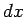along the-axis. Suppose that the difference in electric potential between the final and initial positions of the charge is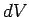. By definition, the change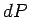in the charge's electric potential energy is given by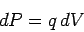(84)

From Eq. (76), the work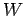which we perform in moving the charge is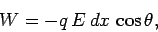(85)

whereis the local electric field-strength, andis the angle subtended between the direction of the field and the-axis. By definition,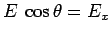, where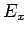is the-component of the local electric field. Energy conservation demands that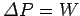(i.e., the increase in the charge's energy matches the work done on the charge), or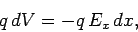(86)

which reduces to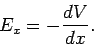(87)

We call the quantity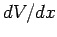the gradient of the electric potential in the-direction. It basically measures how fast the potentialvaries as the coordinateis changed (but the coordinatesandare held constant). Thus, the above formula is saying that the-component of the electric field at a given point in space is equal to minus the local gradient of the electric potential in the-direction.

According to Eq. (87), electric field strength has dimensions of potential difference over length. It follows that the units of electric field are volts per meter (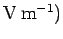. Of course, these new units are entirely equivalent to newtons per coulomb: i.e.,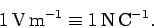(88)

Consider the special case of a uniform-directed electric fieldgenerated by two uniformly charged parallel planes normal to the-axis. It is clear, from Eq. (87), that ifis to be constant between the plates thenmust vary linearly within this region. In fact, it is easily shown that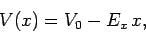(89)

where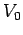is an arbitrary constant. According to Eq. (89), the electric potentialdecreases continuously as we move along the direction of the electric field. Since a positive charge is accelerated in this direction, we conclude that positive charges are accelerated down gradients in the electric potential, in much the same manner as masses fall down gradients of gravitational potential (which is, of course, proportional to height). Likewise, negative charges are accelerated up gradients in the electric potential.

According to Eq. (87), the-component of the electric field is equal to minus the gradient of the electric potential in the-direction. Since there is nothing special about the-direction, analogous rules must exist for the- and-components of the field. These three rules can be combined to give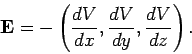(90)

Here, thederivative is taken at constantand, etc. The above expression shows how the electric field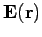, which is a vector field, is related to the electric potential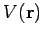, which is a scalar field.

We have seen that electric fields are superposable. That is, the electric field generated by a set of charges distributed in space is simply the vector sum of the electric fields generated by each charge taken separately. Well, if electric fields are superposable, it follows from Eq. (90) that electric potentials must also be superposable. Thus, the electric potential generated by a set of charges distributed in space is just the scalar sum of the potentials generated by each charge taken in isolation. Clearly, it is far easier to determine the potential generated by a set of charges than it is to determine the electric field, since we can sum the potentials generated by the individual charges algebraically, and do not have to worry about their directions (since they have no directions).

Equation (90) looks rather forbidding. Fortunately, however, it is possible to rewrite this equation in a more appealing form. Consider two neighboring pointsand. Suppose that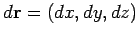is the vector displacement of pointrelative to point. Letbe the difference in electric potential between these two points. Suppose that we travel fromtoby first moving a distancealong the-axis, then moving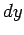along the-axis, and finally moving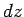along the-axis. The net increase in the electric potentialas we move fromtois simply the sum of the increases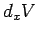as we move along the-axis,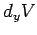as we move along the-axis, and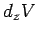as we move along the-axis: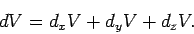(91)

But, according to Eq. (90),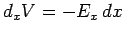, etc. So, we obtain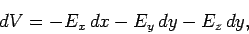(92)

which is equivalent to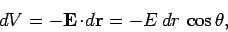(93)

whereis the angle subtended between the vectorand the local electric field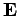. Note thatattains its most negative value when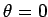. In other words, the direction of the electric field at pointcorresponds to the direction in which the electric potentialdecreases most rapidly. A positive charge placed at pointis accelerated in this direction. Likewise, a negative charge placed atis accelerated in the direction in which the potential increases most rapidly (i.e.,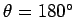). Suppose that we move from pointto a neighboring pointin a direction perpendicular to that of the local electric field (i.e.,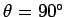). In this case, it follows from Eq. (93) that the pointsandlie at the same electric potential (i.e.,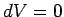). The locus of all the points in the vicinity of pointwhich lie at the same potential asis a plane perpendicular to the direction of the local electric field. More generally, the surfaces of constant electric potential, the so-called equipotential surfaces, exist as a set of non-interlocking surfaces which are everywhere perpendicular to the direction of the electric field. Figure 14 shows the equipotential surfaces (dashed lines) and electric field-lines (solid lines) generated by a positive point charge. In this case, the equipotential surfaces are spheres centred on the charge.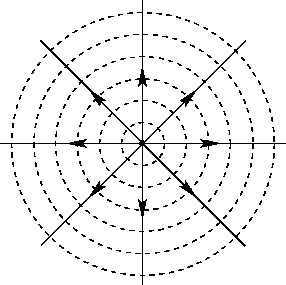In Sect. 4.3, we found that the electric field immediately above the surface of a conductor is directed perpendicular to that surface. Thus, it is clear that the surface of a conductor must correspond to an equipotential surface. In fact, since there is no electric field inside a conductor (and, hence, no gradient in the electric potential), it follows that the whole conductor (i.e., both the surface and the interior) lies at the same electric potential.Next: Electric Potential of a Up: Electric Potential Previous: Electric Potential
Richard Fitzpatrick 2007-07-14